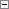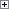Marks a table as being consistent or inconsistent.

Record.CONSISTENT(Consistent)

Parameters

Record

Type: Record

The table that you want to mark.

Consistent

Type: Boolean

The mark to be set on the table.

If this parameter is true, the table is marked as consistent. If this parameter is false, the table is marked as inconsistent.Remarks

Usually this function is only used for accounting routines. If your accounts do not balance, then the accounts are inconsistent. This function makes sure that inconsistent changes are not made in your accounts.

If an attempt is made to commit a write transaction when a table is marked as inconsistent, then a message is displayed and all updates that were made in the write transaction are aborted.Example

A typical example of an inconsistency occurs when the sum of all the entries in a table that contains general ledger entries does not balance (is not equal to zero).

In this example, your application includes a function object that is named ChangeAmount. This function is used to withdraw amounts that were put into the General Ledger Entry table in your application. When an amount is withdrawn from an account, the system is inconsistent until a corresponding amount is put in. The following code example illustrates the ChangeAmount function. The ChangeAmount function has one parameter, Amount, which is a Decimal data type.Copy Code
GLEntry."G/L Account No." := '1000';
GLEntry.Amount := Amount;
GLEntry.INSERT;
Balance := Balance + Amount;
IF Balance = 0 THEN
Consistent := TRUE
ELSE
Consistent := FALSE;
GLEntry.CONSISTENT(Consistent);
END;

The function uses the variable Balance to express the change in balance. For example, when the function is used to withdraw \$100, the Balance variable will reflect this as -\$100.

When you use the function to put in one or more amounts whose total equals +\$100, the Balance variable then equals zero and the table is marked as consistent. This means that if an attempt is made to put in an amount and end the write transaction (commit the change) without withdrawing a corresponding amount, an error occurs and the write transaction is canceled.

Record Data Type